Search by Topic

Filter by: Content type:
Age range:
Challenge level:

There are 215 resultsAge 5 to 11 Challenge Level:

Try out this number trick. What happens with different starting numbers? What do you notice?Subtraction Surprise

Age 7 to 14 Challenge Level:

Try out some calculations. Are you surprised by the results?Making Sense of Positives and Negatives

Age 11 to 14

This article suggests some ways of making sense of calculations involving positive and negative numbers.Take Three Numbers

Age 7 to 11 Challenge Level:

What happens when you add three numbers together? Will your answer be odd or even? How do you know?Dotty Six

Age 5 to 11 Challenge Level:

Dotty Six is a simple dice game that you can adapt in many ways.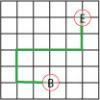Journeys in Numberland

Age 7 to 11 Challenge Level:

Tom and Ben visited Numberland. Use the maps to work out the number of points each of their routes scores.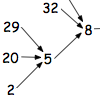Age 7 to 11 Challenge Level:

What happens when you add the digits of a number then multiply the result by 2 and you keep doing this? You could try for different numbers and different rules.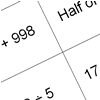How Do You Do It?

Age 7 to 11 Challenge Level:

This group activity will encourage you to share calculation strategies and to think about which strategy might be the most efficient.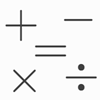Which Symbol?

Age 7 to 11 Challenge Level:

Choose a symbol to put into the number sentence.Nice or Nasty

Age 7 to 14 Challenge Level:

There are nasty versions of this dice game but we'll start with the nice ones...Countdown

Age 7 to 14 Challenge Level:

Here is a chance to play a version of the classic Countdown Game.Magic Vs

Age 7 to 11 Challenge Level:

Can you put the numbers 1-5 in the V shape so that both 'arms' have the same total?Crossed Ends

Age 11 to 14 Challenge Level:

Crosses can be drawn on number grids of various sizes. What do you notice when you add opposite ends?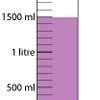Oh! Harry!

Age 7 to 11 Challenge Level:

A group of children are using measuring cylinders but they lose the labels. Can you help relabel them?Weights

Age 11 to 14 Challenge Level:

Different combinations of the weights available allow you to make different totals. Which totals can you make?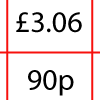How Much Did it Cost?

Age 7 to 11 Challenge Level:

Use your logical-thinking skills to deduce how much Dan's crisps and ice-cream cost altogether.Adding and Subtracting Positive and Negative Numbers

Age 11 to 14

How can we help students make sense of addition and subtraction of negative numbers?Sea Level

Age 7 to 11 Challenge Level:

The picture shows a lighthouse and many underwater creatures. If you know the markings on the lighthouse are 1m apart, can you work out the distances between some of the different creatures?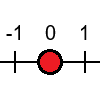Tug Harder!

Age 7 to 11 Challenge Level:

In this game, you can add, subtract, multiply or divide the numbers on the dice. Which will you do so that you get to the end of the number line first?Consecutive Negative Numbers

Age 11 to 14 Challenge Level:

Do you notice anything about the solutions when you add and/or subtract consecutive negative numbers?First Connect Three

Age 7 to 14 Challenge Level:

The idea of this game is to add or subtract the two numbers on the dice and cover the result on the grid, trying to get a line of three. Are there some numbers that are good to aim for?Playing Connect Three

Age 11 to 14 Challenge Level:

In this game the winner is the first to complete a row of three. Are some squares easier to land on than others?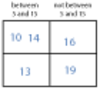More Carroll Diagrams

Age 7 to 11 Challenge Level:

How have the numbers been placed in this Carroll diagram? Which labels would you put on each row and column?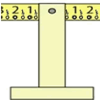Balance of Halves

Age 7 to 11 Challenge Level:

Investigate this balance which is marked in halves. If you had a weight on the left-hand 7, where could you hang two weights on the right to make it balance?Cunning Card Trick

Age 11 to 14 Challenge Level:

Delight your friends with this cunning trick! Can you explain how it works?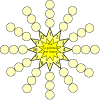The Twelve Pointed Star Game

Age 7 to 11 Challenge Level:

Have a go at this game which involves throwing two dice and adding their totals. Where should you place your counters to be more likely to win?Diagonal Sums

Age 7 to 11 Challenge Level:

In this 100 square, look at the green square which contains the numbers 2, 3, 12 and 13. What is the sum of the numbers that are diagonally opposite each other? What do you notice?Number Differences

Age 7 to 11 Challenge Level:

Place the numbers from 1 to 9 in the squares below so that the difference between joined squares is odd. How many different ways can you do this?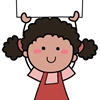Carrying Cards

Age 7 to 11 Challenge Level:

These sixteen children are standing in four lines of four, one behind the other. They are each holding a card with a number on it. Can you work out the missing numbers?Number Pyramids

Age 11 to 14 Challenge Level:

Try entering different sets of numbers in the number pyramids. How does the total at the top change?A Mixed-up Clock

Age 7 to 11 Challenge Level:

There is a clock-face where the numbers have become all mixed up. Can you find out where all the numbers have got to from these ten statements?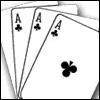Amazing Card Trick

Age 11 to 14 Challenge Level:

How is it possible to predict the card?The Deca Tree

Age 7 to 11 Challenge Level:

Find out what a Deca Tree is and then work out how many leaves there will be after the woodcutter has cut off a trunk, a branch, a twig and a leaf.A Square of Numbers

Age 7 to 11 Challenge Level:

Can you put the numbers 1 to 8 into the circles so that the four calculations are correct?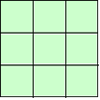1, 2, 3 Magic Square

Age 7 to 11 Challenge Level:

Arrange three 1s, three 2s and three 3s in this square so that every row, column and diagonal adds to the same total.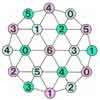Totality

Age 5 to 11 Challenge Level:

This is an adding game for two players.Cubes Within Cubes

Age 7 to 14 Challenge Level:

We start with one yellow cube and build around it to make a 3x3x3 cube with red cubes. Then we build around that red cube with blue cubes and so on. How many cubes of each colour have we used?Two Primes Make One Square

Age 7 to 11 Challenge Level:

Can you make square numbers by adding two prime numbers together?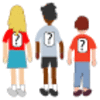One Wasn't Square

Age 7 to 11 Challenge Level:

Mrs Morgan, the class's teacher, pinned numbers onto the backs of three children. Use the information to find out what the three numbers were.Abundant Numbers

Age 7 to 11 Challenge Level:

48 is called an abundant number because it is less than the sum of its factors (without itself). Can you find some more abundant numbers?Zios and Zepts

Age 7 to 11 Challenge Level:

On the planet Vuv there are two sorts of creatures. The Zios have 3 legs and the Zepts have 7 legs. The great planetary explorer Nico counted 52 legs. How many Zios and how many Zepts were there?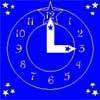Watch the Clock

Age 7 to 11 Challenge Level:

During the third hour after midnight the hands on a clock point in the same direction (so one hand is over the top of the other). At what time, to the nearest second, does this happen?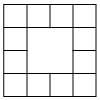Prison Cells

Age 7 to 11 Challenge Level:

There are 78 prisoners in a square cell block of twelve cells. The clever prison warder arranged them so there were 25 along each wall of the prison block. How did he do it?Number Daisy

Age 11 to 14 Challenge Level:

Can you find six numbers to go in the Daisy from which you can make all the numbers from 1 to a number bigger than 25?Two and Two

Age 11 to 14 Challenge Level:

How many solutions can you find to this sum? Each of the different letters stands for a different number.Neighbours

Age 7 to 11 Challenge Level:

In a square in which the houses are evenly spaced, numbers 3 and 10 are opposite each other. What is the smallest and what is the largest possible number of houses in the square?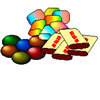The Puzzling Sweet Shop

Age 7 to 11 Challenge Level:

There were chews for 2p, mini eggs for 3p, Chocko bars for 5p and lollypops for 7p in the sweet shop. What could each of the children buy with their money?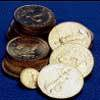Five Coins

Age 7 to 11 Challenge Level:

Ben has five coins in his pocket. How much money might he have?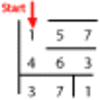Maze 100

Age 7 to 11 Challenge Level:

Can you go through this maze so that the numbers you pass add to exactly 100?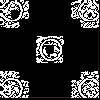Doplication

Age 7 to 11 Challenge Level:

We can arrange dots in a similar way to the 5 on a dice and they usually sit quite well into a rectangular shape. How many altogether in this 3 by 5? What happens for other sizes?